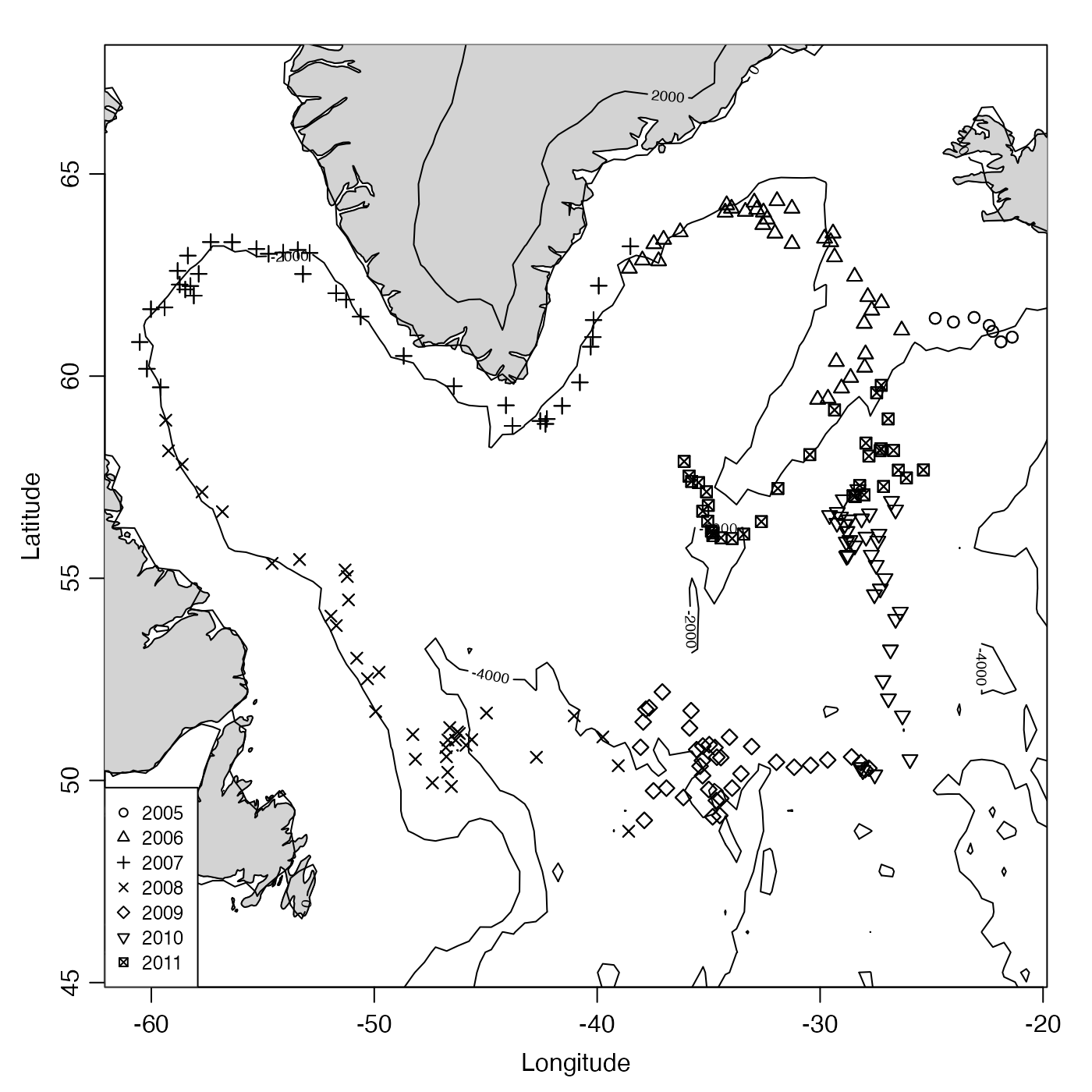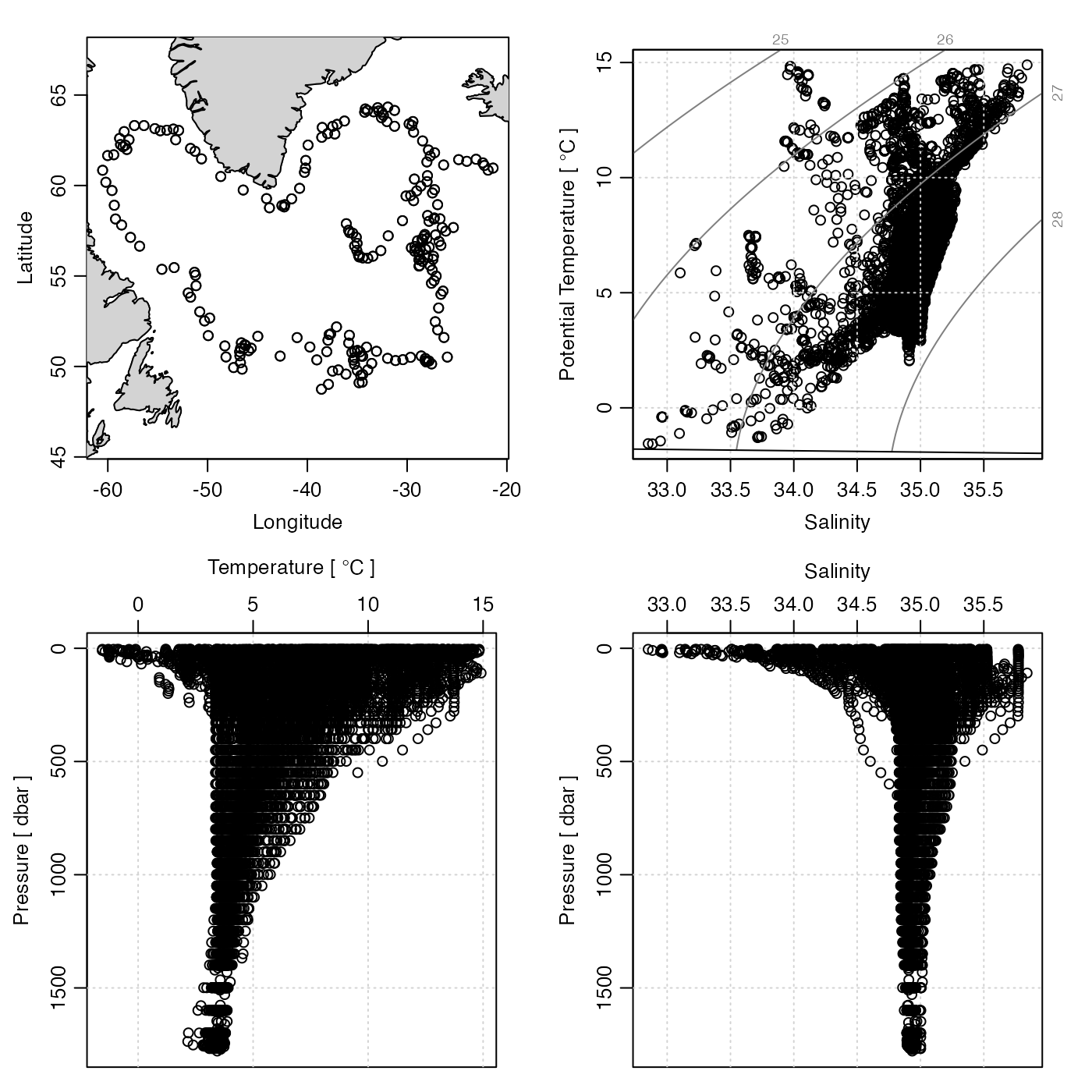Plot a summary diagram for argo data.

# S4 method for argo
plot(
x,
which = 1,
level,
coastline = c("best", "coastlineWorld", "coastlineWorldMedium", "coastlineWorldFine",
"none"),
cex = 1,
pch = 1,
type = "p",
col = 1,
fill = FALSE,
projection = NULL,
mgp = getOption("oceMgp"),
mar = c(mgp + 1.5, mgp + 1.5, 1.5, 1.5),
tformat,
debug = getOption("oceDebug"),
...
)

## Arguments

x

an argo object.

which

list of desired plot types, one of the following. Note that oce.pmatch() is used to try to complete partial character matches, and that an error will occur if the match is not complete (e.g. "salinity" matches to both "salinity ts" and "salinity profile".).

• which=1, which="trajectory" or which="map" gives a plot of the argo trajectory, with the coastline, if one is provided.

• which=2 or "salinity ts" gives a time series of salinity at the indicated level(s)

• which=3 or "temperature ts" gives a time series of temperature at the indicated level(s)

• which=4 or "TS" gives a TS diagram at the indicated level(s)

• which=5 or "salinity profile" gives a salinity profile of all the data (with S and p trimmed to the 1 and 99 percentiles)

• which=6 or "temperature profile" gives a temperature profile (with T and p trimmed to the 1 and 99 percentiles)

level

depth pseudo-level to plot, for which=2 and higher. May be an integer, in which case it refers to an index of depth (1 being the top) or it may be the string "all" which means to plot all data.

coastline

character string giving the coastline to be used in an Argo-location map, or "best" to pick the one with highest resolution, or "none" to avoid drawing the coastline.

cex

size of plotting symbols to be used if type="p".

pch

type of plotting symbols to be used if type="p".

type

plot type, either "l" or "p".

col

optional list of colors for plotting.

fill

Either a logical, indicating whether to fill the land with light-gray, or a color name. Owing to problems with some projections, the default is not to fill.

projection

indication of the projection to be used in trajectory maps. If this is NULL, no projection is used, although the plot aspect ratio will be set to yield zero shape distortion at the mean float latitude. If projection="automatic", then one of two projections is used: stereopolar (i.e. "+proj=stere +lon_0=X" where X is the mean longitude), or Mercator (i.e. "+proj=merc") otherwise. Otherwise, projection must be a character string specifying a projection in the notation used by oceProject() and mapPlot().

mgp

3-element numerical vector to use for par(mgp), and also for par(mar), computed from this. The default is tighter than the R default, in order to use more space for the data and less for the axes.

mar

value to be used with par("mar").

tformat

optional argument passed to oce.plot.ts(), for plot types that call that function. (See strptime() for the format used.)

debug

debugging flag.

...

optional arguments passed to plotting functions.

## Value

None.

Other things related to argo data: [[,argo-method, [[<-,argo-method, argo-class, argoGrid(), argoNames2oceNames(), argo, as.argo(), handleFlags,argo-method, read.argo.copernicus(), read.argo(), subset,argo-method, summary,argo-method

Other functions that plot oce data: download.amsr(), plot,adp-method, plot,adv-method, plot,amsr-method, plot,bremen-method, plot,cm-method, plot,coastline-method, plot,ctd-method, plot,gps-method, plot,ladp-method, plot,landsat-method, plot,lisst-method, plot,lobo-method, plot,met-method, plot,odf-method, plot,rsk-method, plot,satellite-method, plot,sealevel-method, plot,section-method, plot,tidem-method, plot,topo-method, plot,windrose-method, plot,xbt-method, plotProfile(), plotScan(), plotTS(), tidem-class

Dan Kelley

## Examples

library(oce)
data(argo)
tc <- cut(argo[["time"]], "year")
# Example 1: plot map, which reveals float trajectory.
plot(argo, pch = as.integer(tc))
year <- substr(levels(tc), 1, 4)
data(topoWorld)
contour(topoWorld[["longitude"]], topoWorld[["latitude"]],
topoWorld[["z"]],